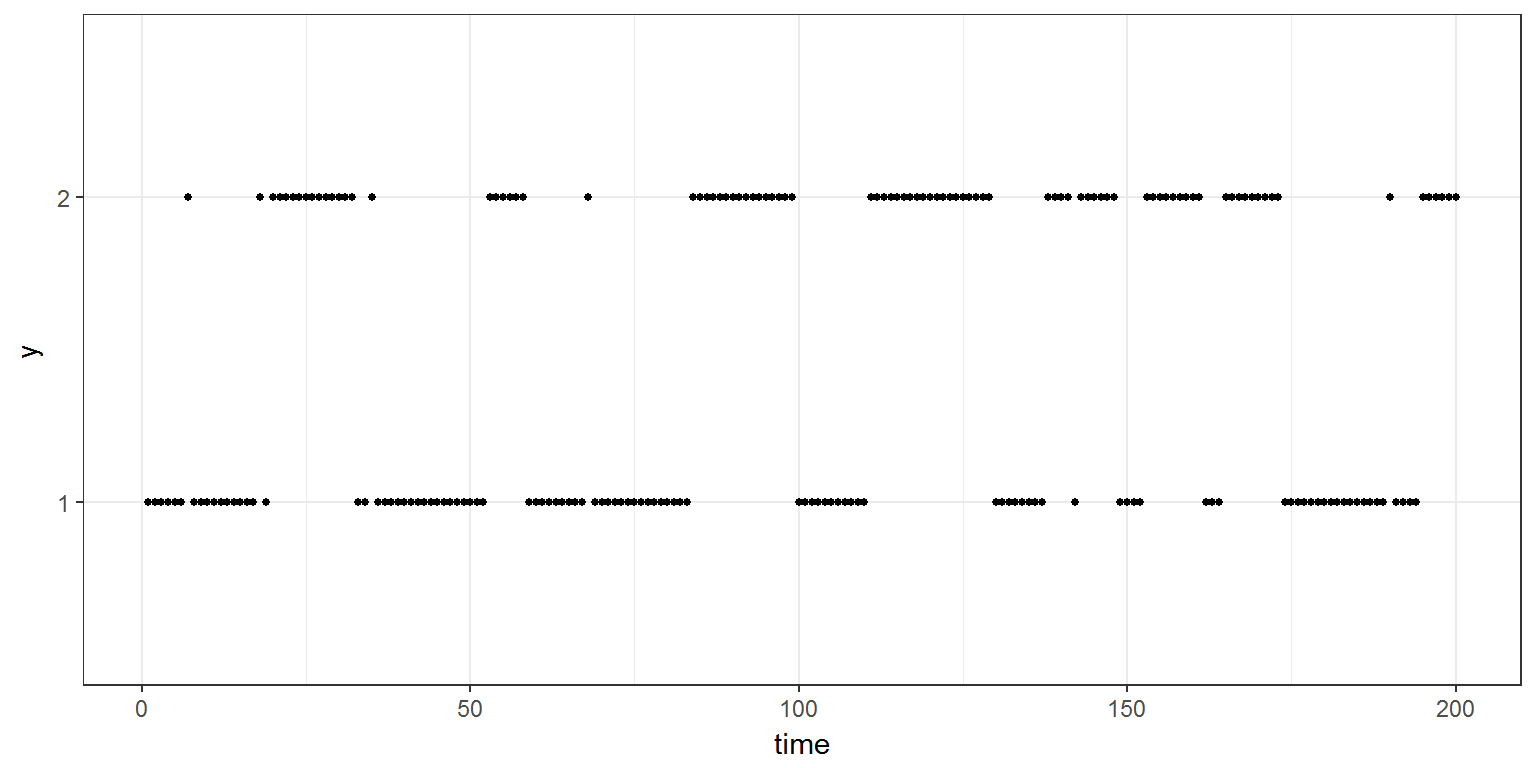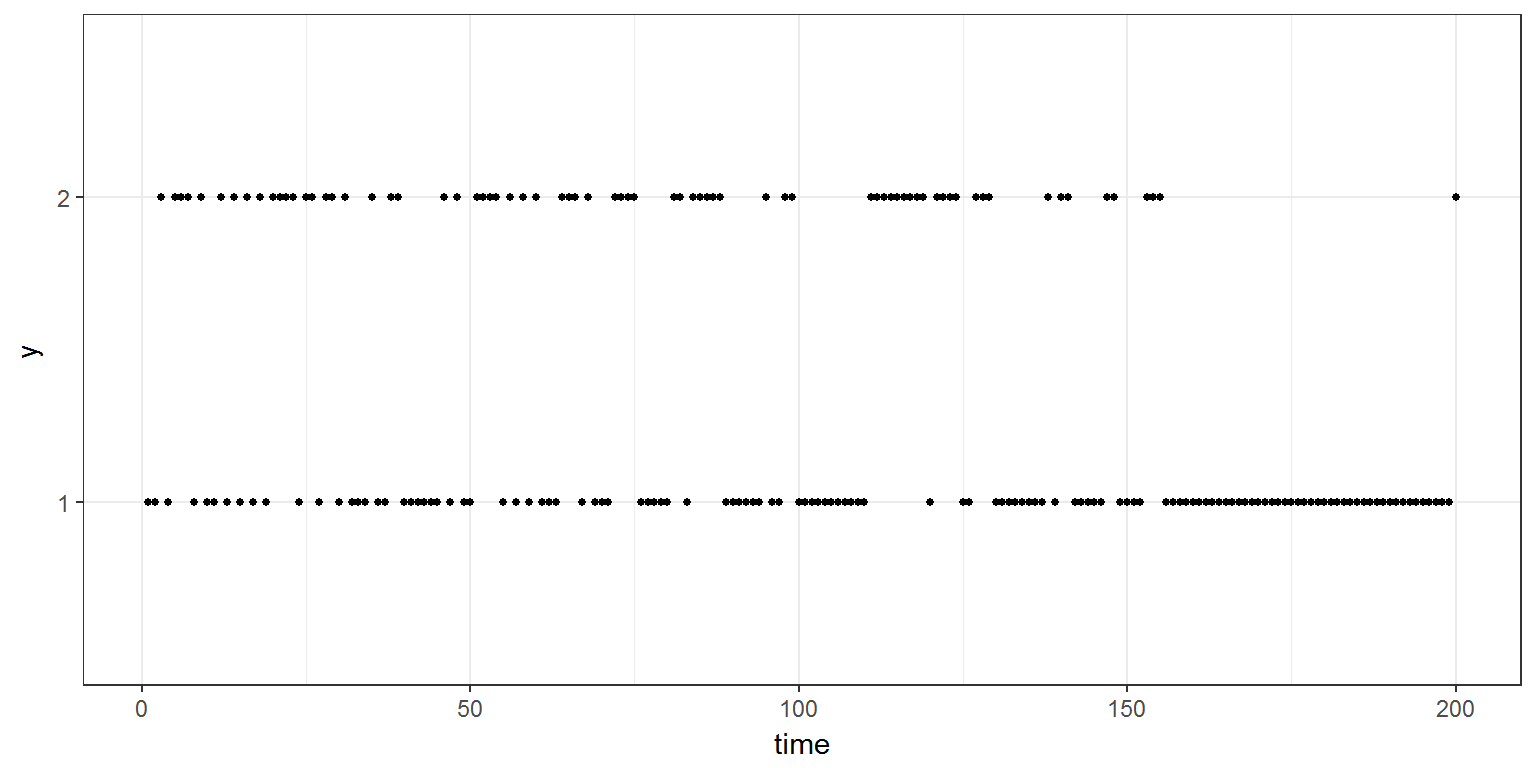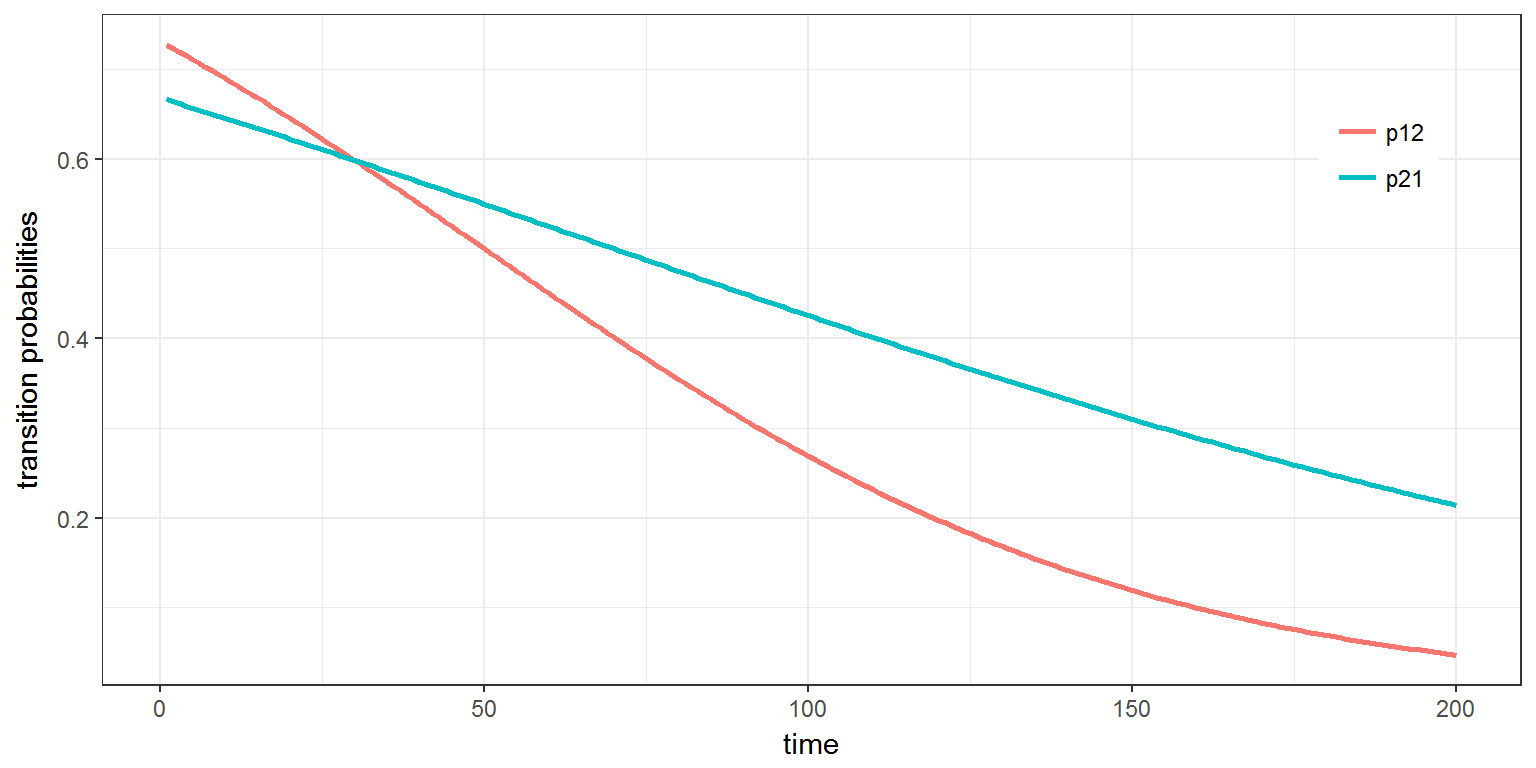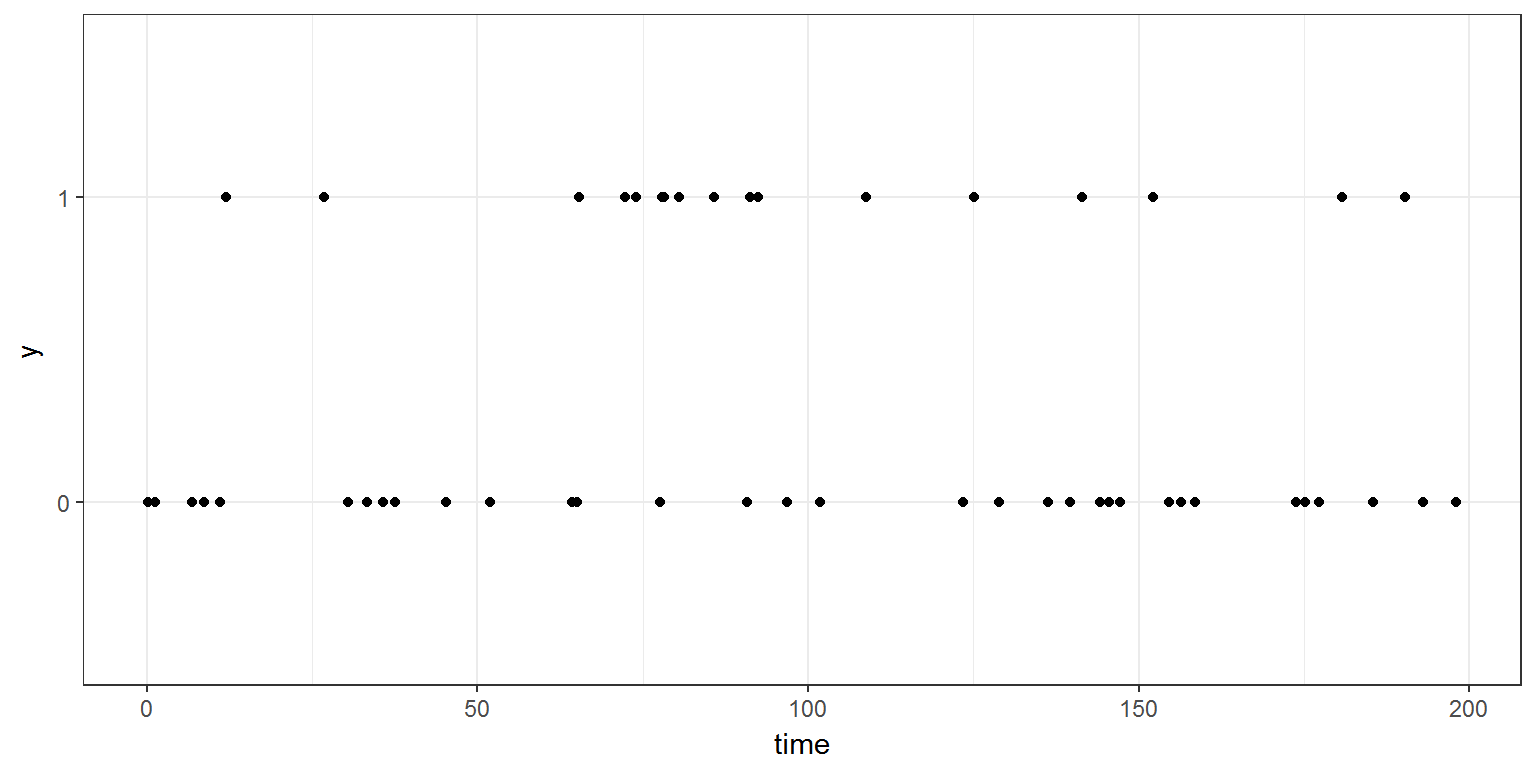R script: markovian.R

Mlxtran code: model/markovianA.txt ; model/markovianB.txt ; model/markovianC.txt

$$\newcommand{\esp}{\mathbb{E}\left(#1\right)} \newcommand{\var}{\mbox{Var}\left(#1\right)} \newcommand{\deriv}{\dot{#1}(t)} \newcommand{\prob}{ \mathbb{P}\!(#1)} \newcommand{\eqdef}{\mathop{=}\limits^{\mathrm{def}}} \newcommand{\by}{\boldsymbol{y}} \newcommand{\bc}{\boldsymbol{c}} \newcommand{\bpsi}{\boldsymbol{\psi}} \def\pmacro{\texttt{p}} \def\like{{\cal L}} \def\llike{{\cal LL}} \def\logit{{\rm logit}} \def\probit{{\rm probit}} \def\one{{\rm 1\!I}} \def\iid{\mathop{\sim}_{\rm i.i.d.}} \def\simh0{\mathop{\sim}_{H_0}} \def\df{\texttt{df}} \def\res{e} \def\xomega{x} \newcommand{\argmin}{{\rm arg}\min_{#1}} \newcommand{\argmax}{{\rm arg}\max_{#1}} \newcommand{\Rset}{\mbox{\mathbb{R}}} \def\param{\theta} \def\setparam{\Theta} \def\xnew{x_{\rm new}} \def\fnew{f_{\rm new}} \def\ynew{y_{\rm new}} \def\nnew{n_{\rm new}} \def\enew{e_{\rm new}} \def\Xnew{X_{\rm new}} \def\hfnew{\widehat{\fnew}} \def\degree{m} \def\nbeta{d} \newcommand{\limite}{\mathop{\longrightarrow}\limits_{#1}} \def\ka{k{a}} \def\ska{k{\scriptscriptstyle a}} \def\kel{k{e}} \def\skel{k{\scriptscriptstyle e}} \def\cl{C{\small l}} \def\Tlag{T\hspace{-0.1em}{lag}} \def\sTlag{T\hspace{-0.07em}{\scriptscriptstyle lag}} \def\Tk{T\hspace{-0.1em}{k0}} \def\sTk{T\hspace{-0.07em}{\scriptscriptstyle k0}} \def\thalf{t{1/2}} \newcommand{\Dphi}{\partial_\pphi #1} \def\asigma{a} \def\pphi{\psi} \newcommand{\stheta}{{\theta^\star}} \newcommand{\htheta}{{\widehat{\theta}}}$$

# 1 Introduction

For the sake of simplicity, we will assume here that the observations $$(y_{j})$$ take their values in $$\{1, 2, \ldots, K\}$$.

We have so far assumed that the categorical observations $$(y_{j},\,j=1,2,\ldots,n)$$ are independent. It is however possible to introduce dependence between observations from the same individual by assuming that $$(y_{j},\,j=1,2,\ldots,n)$$ forms a Markov chain. For instance, a Markov chain with memory 1 assumes that all that is required from the past to determine the distribution of $$y_{j}$$ is the value of the previous observation $$y_{j-1}$$, i.e., for all $$k=1,2,\ldots ,K$$, $\prob{y_{j} = k\,|\,y_{j-1}, y_{j-2}, y_{j-3},\ldots} = \prob{y_{j} = k | y_{j-1}}.$

## 1.1 Discrete-time Markov chain

If observation times are regularly spaced (constant length of time between successive observations), we can consider the observations $$(y_{j},\,j=1,2,\ldots,n)$$ to be a discrete-time Markov chain. Here, the probability distribution of the sequence $$(y_{j})$$ is defined by

• The distribution $$\pi = (\pi_{k} , k=1,2,\ldots,K)$$ of the first observation $$y_{1}$$: $\pi_{k} = \prob{y_{1} = k } .$
• The sequence of transition matrices $$(Q_{j},\, j=2,3,\ldots)$$ where for each $$j$$, $$Q_{j} = (q_{j,\ell,k}, 1\leq \ell,k \leq K)$$ is a matrix of size $$K \times K$$ such that

\begin{align} q_{j,\ell,k} &= \prob{y_{j} = k |\, y_{j-1}=\ell} \quad \text{ for all } \,\, 1\leq \ell,k \leq K,\\ \sum_{k=1}^{K}q_{j,\ell,k} &= 1 \quad \text{ for all }\,\, 1\leq \ell \leq K. \end{align}

## 1.2 Continuous-time Markov chain

The previous situation can be extended to the case where time intervals between observations are irregular by modeling the sequence of states as a continuous-time Markov process. The difference is that rather than transitioning to a new (possibly the same) state at each time step, the system remains in the current state for some random amount of time before transitioning.

This process is now characterized by transition rates instead of transition probabilities: $\prob{y(t+h) = k\,|\,y(t)=\ell } = h \, \rho_{\ell k}(t) + o(h),\qquad k \neq \ell .$

The probability that no transition happens between $$t$$ and $$t+h$$ is $\prob{y(s) = \ell, \forall s\in(t, t+h) \ | \ y(t)=\ell } = e^{h \, \rho_{\ell \ell}(t)} .$ Furthermore, for any time $$t$$, the transition rates $$(\rho_{\ell,k}(t))$$ satisfy for any $$1\leq \ell \leq K$$, $\sum_{k=1}^K \rho_{\ell k}(t) = 0.$

# 2 Examples

## 2.1 Example 1: Homogeneous discrete-time Markov chain

We consider a very simple Markov model with 2 states and where the transition probabilities remain constant over time:

\begin{aligned} \prob{y_j =2 | y_{j-1} = 1} & = 1 - \prob{y_j =1 | y_{j-1} = 1} = p_{12} \\ \prob{y_j =1 | y_{j-1} = 2} & = 1 - \prob{y_j =2 | y_{j-1} = 2} = p_{21} \end{aligned}

This model is implemented in the file categoricalA.txt:


[LONGITUDINAL]
input = {p12, p21}

DEFINITION:
y = {type       = categorical
categories = {1, 2}
dependence = Markov
P(y=2 |y_p=1)=p12
P(y=1 |y_p=2)=p21}


We can now generate a sequence of $$n=200$$ observations with this model using Simulx.

seed <- 12345
p    <- c(p12=0.2, p21=0.15)
y    <- list(name='y', time=seq(1, 200))
res1  <- simulx(model     = 'model/markovianA.txt',
parameter = p,
output    = y,
settings  = list(seed=seed))

ggplot(data=res1y) + geom_point(aes(x=time, y=y),size=1)## 2.2 Example 2: Non homogeneous discrete-time Markov chain The transition probabilities change with time in this second example. These cumulative logits are defined as follow: \begin{aligned} {\rm logit}(\prob{y_j =2 | y_{j-1} = 1}) & = a + b\, t_j \\ {\rm logit}(\prob{y_j =1 | y_{j-1} = 2}) & = c + d\, t_j \end{aligned} This model is implemented in the file categoricalB.txt:  [LONGITUDINAL] input = {a, b, c, d} EQUATION: l12 = a+b*t l21 = c+d*t p12 = 1/(1+exp(-l12)) p21 = 1/(1+exp(-l21)) DEFINITION: y = {type = categorical categories = {1, 2} dependence = Markov logit(P(y=2 |y_p=1))=l12 logit(P(y=1 |y_p=2))=l21}  We can generate a new sequence of $$n=200$$ observations with this new model. p <- c(a=1,b=-0.02,c=0.7,d=-0.01) f <- list(name=c('p12','p21'), time=seq(1, 200)) res2 <- simulx(model = 'model/markovianB.txt', parameter = p, output = list(y,f), settings = list(seed=seed)) ggplot(res2y) + geom_point(aes(x=time, y=y),size=1)and we can plot the transition probabilities $$p_{12}(t)$$ and $$p_{21}(t)$$ defined in the model.

library(reshape2)
r <- melt(merge(res2$p12,res2$p21) ,  id = 'time', variable.name = 'f')
print(ggplot(r, aes(time,value)) + geom_line(aes(colour = f),size=1) +
ylab('transition probabilities') + guides(colour=guide_legend(title=NULL)) +
theme(legend.position=c(.9, .8)))## 2.3 Example 3: Continuous-time Markov chain

We now consider a continuous-time Markov chain: the observation times are irregularly distributed between 0 and 200.

The transition rates are constant over time in the model implemented in the file categoricalC.txt:


[LONGITUDINAL]
input = {q01, q10}

DEFINITION:
y = {type       = categorical
categories = {0, 1}
dependence = Markov
transitionRate(0,1) = q01
transitionRate(1,0) = q10
}

50 time points are randomly distributed between 0 and 200 in this example.

q    <- c(q01=0.8, q10=1)
y    <- list(name='y', time=sort(runif(50,min=0,max=200)))
res3  <- simulx(model     = 'model/markovianC.txt',
parameter = q,
output    = y,
settings  = list(seed=12345))

plot(ggplot(data=res3\$y) + geom_point(aes(x=time, y=y)))